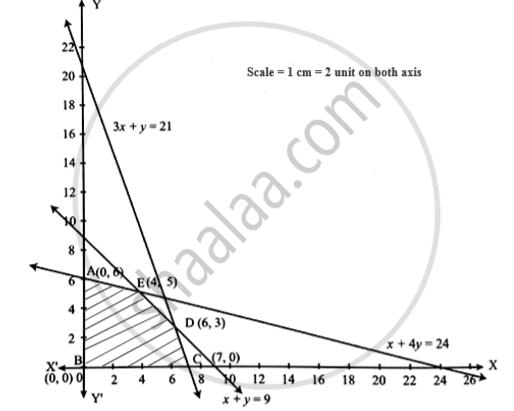Advertisement Remove all ads

# Maximize: 3 5 Subjectto - Mathematics and Statistics

Graph

Maximize: z = 3x + 5y  Subject to

x +4y ≤ 24                3x + y  ≤ 21

x + y ≤ 9                     x ≥ 0 , y ≥0

Advertisement Remove all ads

#### Solution

 Inequation Point on x-axis Point on y-axis Feasible Region x +4y ≤ 24 (24,0) (0,6) Origin side 3x +y ≤ 21 (7,0) (0,21) Origin side x + y ≤9 (9,0) (0,9) Origin sideFrom the figure common feasible region is ABCDEA
E is the point of intersection of  x + y = 9 and x + 4y =24
Solving them we get E(4,5)
D is the point of intersection of  x + y = 9 and 3x +y = 21
Solving them we get D(6,3)

 End Point Value of z = 3x +5y A(0,6) 0 +30= 30 B(0,0) 0 + 0 = 0 C(7,0) 21 + 0 = 21 D(6,3) 18 +1 5 = 33 E(4,5) 12 +25 = 37

∴ z is maximum 37 at the point (4, 5)

Is there an error in this question or solution?
Advertisement Remove all ads

#### APPEARS IN

Advertisement Remove all ads

#### Video TutorialsVIEW ALL 

Advertisement Remove all ads
Share
Notifications

View all notifications

Forgot password?